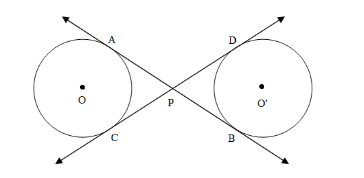QuestionAnswers

# AB and CD are common tangents to two circles of equal radii. Prove that AB = CD.

Hint: In order to solve such a problem use the property of tangents to the circle which states that lengths of two different tangents drawn from the same point to the circle are the same.

Given: Two circles with centre O and O’. AB and CD are common tangents to the circles which intersect in P. As shown in the figure.To prove AB = CD
As we know that the length of the tangents drawn from an external point to the circle are equal.
For Circle with center O and external point P. We have:
$AP = PC$ ------ (1)
For Circle with center O’ and external point P. We have:
$PB = PD$ ------ (2)
Now finally adding equation (1) and equation (2) we get:
$\Rightarrow AP + PB = PC + PD \\ \Rightarrow AB = CD \\$
Hence, we have proved $AB = CD$

Note: In order to solve such a type of problem, it is very important to remember the properties of the tangents of the circle. It is also important to visualize the figure and recognize the point where this property can be used.